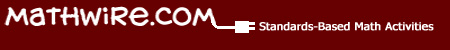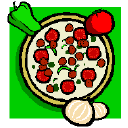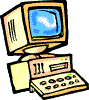# FractionsVirtual manipulatives allow students to manipulate fraction models and provide immediate feedback, in many cases, so that students "see" equivalence, comparison, etc. These models are also very effectively used by teachers who have access to an interactive whiteboard or large classroom monitor for instructional activities.

•Fraction Bars
•Fraction Pieces use the circular area model.
•Fractions: Parts of a Whole connects the shaded picture and the fraction.
•Fractions: Naming requires students to write a fraction for the given shaded picture.
•Fractions: Visualizing assesses student understanding of fraction numerators and denominators by requiring students to first create the correct number of pieces, then shade in the appropriate number to match the given fraction.
•Fraction Model I presents picture, fraction, plus equivalent decimal and percent.

Lesson Plans include introduction, lesson plans, student handouts and assessment for these fraction lessons.•Who Wants Pizza? develops fractions through the scenario of sharing pizza.
•No Matter What Shape Your Fractions Are In uses pattern blocks to develop fraction concepts.
•The Sweet Tooth Factory is a fraction unit that could be used as a math center during fraction units.

Fraction Games provide extended practice of fraction concepts.•Fresh Baked Fractions challenges students to identify the fraction that is not equivalent to the other three.
•Fraction Games offers games to practice many fractional skills.
•Fraction Frenzy, from Learning Planet, challenges students to identify the equivalent fraction pairs before time runs out on each level of this multi-level game.
•Fraction Match is a concentration game in which students match the fraction to the picture.
•Fraction Track Game from NCTM challenges students to apply working knowledge of equivalent fractions to win the game.
•Fraction Game is a one-person version of the Fraction Track Game.
•Fraction Monkeys requires students to hang fraction monkeys at the correct place on the number line.
•Fraction Flags: Halves and Quarters allows students to paint flag sections to match the given colors and fractions.
•Melvin's Make a Match requires students to match the picture bottles to the fraction bottles.
•Tony Fraction: Pizza Game challenges students to assemble a pizza with the correct fractional toppings before time runs out.